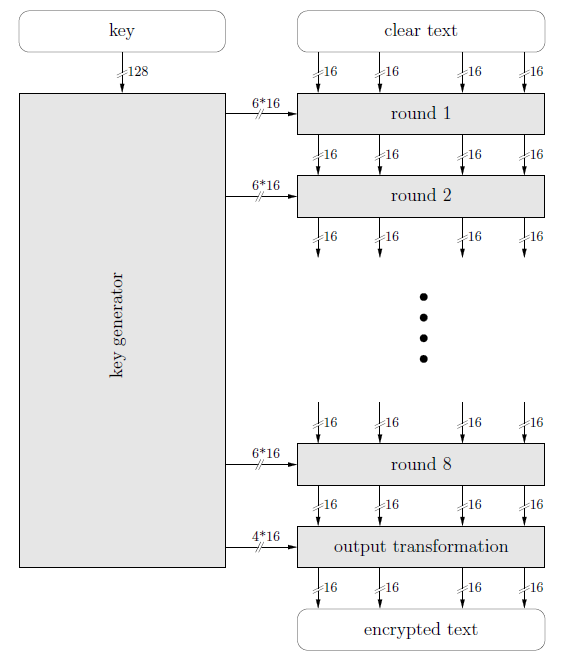IDEA – International Data Encryption Algorithm. It is patent protected to prevent fraud and privacy.It was meant to be a replacement for the Data Encryption Standard. It is considered among the best known publicly algorithms . It is a block cipher that takes input of 64 bit and key used is of 128 bit from which we derive 52 subkeys that is used in the algorithm.

Idea perform 8 identical round for encryption in which 6 different subkeys are used and at last four keys are used for output transformation. IDEA was used in Pretty Good Privacy.

The process performed in each rounds are:

(PT1, PT2, PT3 and PT4 are the four parts (block) of Plain text that is an input for the rounds of IDEA

Each PT is of 16-bit that in total 64-bit of plain text)

1) Multiply PT1 and Key1

2) Add PT2 and Key 2

3) Add PT3 and Key 3

4) Multiply PT4 and Key K4

5) result of Step 1 XOR result of step 3

6) result of Step 2 XOR result of step 4

7) Multiply step 5 with Key 5

8) Add result of step 6 and step 7

9) Multiply result of step 8 with Key 6.

10) Add result of step 7 and step 9.

11) result of steps 1 XOR result of step 9.

12) result of steps 3 XOR result of step 9.

13) result of steps 2 XOR result of step 10.

14) result of steps 4 XOR result of step 10.

OUTPUT TRANSFORMATION :

1) Multiply R1 with Key 49.

2) Add R2 and Key 50.

3) Add R3 and Key 51.

4) Multiply R4 and Key 52.

(RI, R2, R3 AND R4 are the four blocks (part) Plain text after the complete 8 rounds , which are the inputs for the output transformation resulting in cipher text.)